• 该PPT讲了求最短路算法SPFA,Bellman-Ford和Floyed-Warshall算法，还拓展了差分约束。十分适合初学者用
• 最 短 路 问 题 一问题的提法及应用背景 1 问题的提法 寻求网络中两点间的最短路就是 寻求连接这两个点的边的 总权数最小的 通路 注意 在有向图中通路 开的初等链中所有的弧应是 首 尾相连 的 2 应用背景 管道铺设...
• c#写了一个最短路算法，希望能够对他人有用.......................................................................................................................
• 基于MATLAB软件，在复杂网络中计算最短路径的函数，文件一共2个复杂网络 最短路径
• 蚁群算法求解最短路，MATLAB程序，可用于路径规划等，有详细说明
• 采用matlab语言编写高效程序，实现快速又高效的最短路和次短路算法
• 对于K最短路问题，首先找出两点之间的所有路径，然后利用K最短路算法，将最短路、次短路、第三最短路等计算出来，存入数组中。该matlab程序具有很好的通用性，希望对大家有用。 说明：findpath.m文件可计算出任意两...matlab
• matlab编写迪杰斯特拉算法求解求最短路问题,文件是程序源代码
• 基于MATLAB求解最短路问题，dijkstra最短路径算法的详细解读。Matlab
• % 离散优化 % *enum - 枚举法 % *monte - 蒙特卡洛法 ...% *minroute - 最短路dijkstra算法 % *krusk - 最小生成树kruskal算法mex程序 % *dijkstra - 最短路dijkstra算法mex程序 % *dynprog - 动态规划MATLAB
• lingo最短路问题，最短路问题代码，lingo模型常用题型
• lingo算法实现单源单宿最短路问题代码 线性规划的学习内容 有完整代码，包括带整数约束的
• 最短路计数最短路计数最短路计数最短路计数最短路计数最短路计数最短路计数
• 管理运筹学试题及答案(线性规划,运输问题,动态规划,最短路最大流).pdf
• 数学建模必备的最短路径问题，愿同学们取的好成绩，有代码有课件
• 最 短 路 问 题 一问题的提法及应用背景 1问题的提法寻求网络中两点间的最短路就是寻求连接这两个点的边的总权数最小的通路注意在有向图中通路开的初等链中所有的弧应是首尾相连的 2应用背景管道铺设线路安排厂区...
• 最短路算法 ;1D氏标号法Dijkstra 1求解思路从始点出发逐步顺序地向外探寻每向外延伸一步都要求是最短的 ;3选用符号的意义 P 标号Permanent固定/永久性标号 从始点到该标号点的最短路权 T 标号Temporary临时性标号 ...
• Floyd最短路matlab算法，经典的运筹学问题。hiuhoojjljljpiojupo
• 论文研究-无向正权网络最短路模型的建立和理论分析.pdf, 路径问题是运筹学的重要分支, 更是图论学科成立的奠基问题.针对无向网络中的路径问题, 首先, 建立了无向正权...
• 模拟退火算法求最短路在数学建模也十分常见，本文给的代码有详细注释，小白也可以轻松明白。
• 提出了点和边都带有成本约束的最短路问题，证明了该问题是NP-完全的。建立了这类问题的数学规划模型，并采用拉格朗日松弛算法对模型进行求解，给出了次梯度优化求解算法的一般步骤。考虑到算法在实际求解过程中收敛...
• 函数m文件 函数的参数是链接矩阵 起始路点，终点 函数输出为最短路的长度和路径，自身为0 无路径为无穷大
• k则最短路算法文献，理论严密算法中的删除路径算法，santos。
• 文章目录最短路问题分类朴素Dijkstra算法 最短路问题分类 最短路算法知识结构图 注：n为顶点数，m为边数 每种不同的情况都有相应最适合的算法，但不用拘泥于一定要用某个算法。 单源最短路：求一个点到其他所有点的...


文章目录
最短路问题分类单源最短路朴素Dijkstra算法堆优化版DijkstraBellman-Ford算法SPFA算法**SPFA算法判断负环**

多源汇最短路Floyd算法

最短路问题分类
最短路算法知识结构图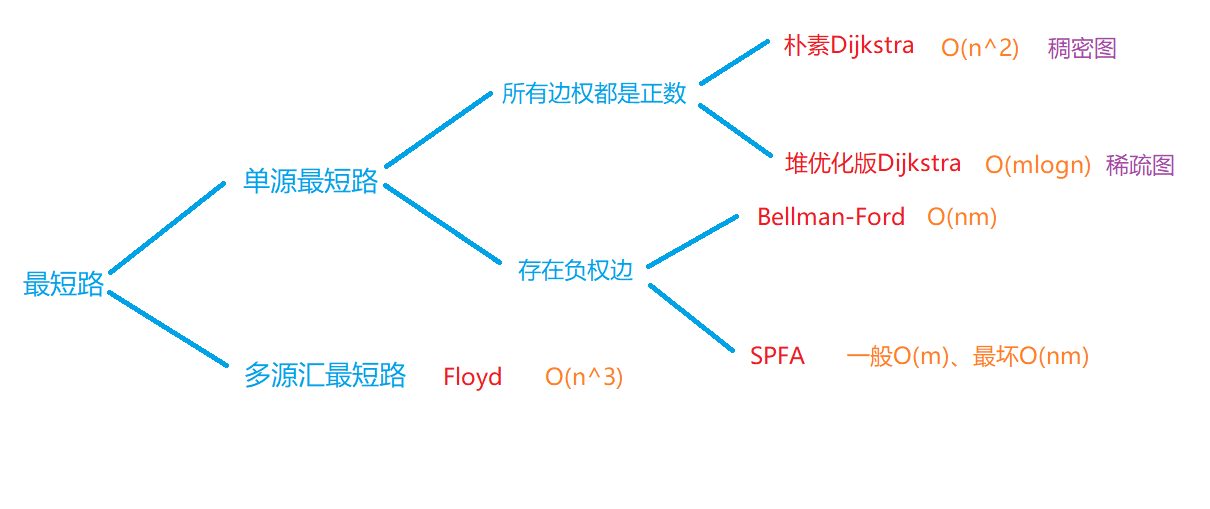注：n为顶点数，m为边数 每种不同的情况都有相应最适合的算法，但不用拘泥于一定要用某个算法。 单源最短路：求一个点到其他所有点的最短距离 多源汇最短路：源 指 起点，汇 指 终点。任意两点间的最短距离（起点、终点不确定） 稠密图：m至少和n^2大致是一个级别 （稠密图用邻接矩阵存储） 稀疏图：m至少和n大致是一个级别 （稀疏图用邻接表存储） 重点：建图，如何把原问题抽象成一个最短路问题，如何定义点和边。
单源最短路
朴素Dijkstra算法
实现步骤： 1.初始化距离 dist = 0，dist[i] = + ∞ （dist数组表示起点到i点的距离） 2.for循环 1~n循环n次 （s：当前已确定最短距离的点） ①找到不在s中的距离起点最近的点，赋给t O(n^2) ②把t加到s中去 O(n) ③用t来更新其他所有点的距离 O(m)（看从1号点<起点>到x的距离是否大于到t的距离，即dist[x] > dist[t] 如果是，则用t来更新) 找到没有确定最短路且距离起点最近的点，并通过这个点更新其他点到起点的最短距离（即以这个点为过渡点） 每次循环都可以确定一个点的最短距离，循环n次就可以确定每个点到起点的最短距离了。 模板
int g[N][N];  // 存储每条边
int dist[N];  // 存储1号点到每个点的最短距离
bool st[N];   // 存储每个点的最短路是否已经确定

// 求1号点到n号点的最短路，如果不存在则返回-1
int dijkstra()
{
memset(dist, 0x3f, sizeof dist);
dist = 0;

for (int i = 0; i < n - 1; i ++ )
{
int t = -1;     // 在还未确定最短路的点中，寻找距离最小的点
for (int j = 1; j <= n; j ++ )
if (!st[j] && (t == -1 || dist[t] > dist[j]))
t = j;

// 用t更新其他点的距离
for (int j = 1; j <= n; j ++ )
dist[j] = min(dist[j], dist[t] + g[t][j]);

st[t] = true;
}

if (dist[n] == 0x3f3f3f3f) return -1;
return dist[n];
}

典例——AcWing 849.Dijkstra求最短路I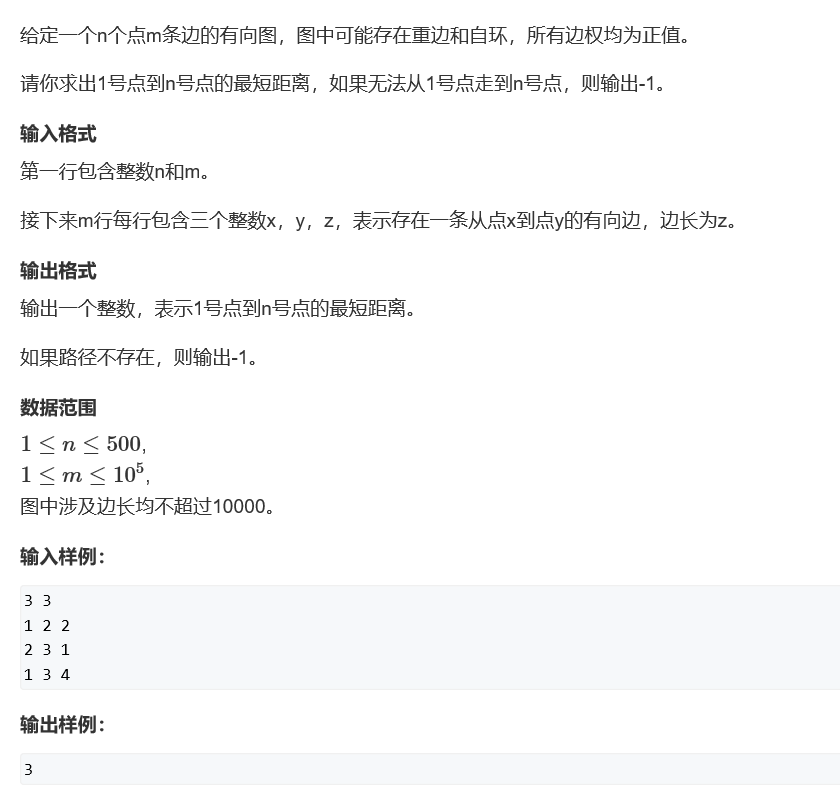#include <iostream>
#include <cstring>
#include <algorithm>

using namespace std;

const int N = 510;

int n,m;
int g[N][N];  //邻接矩阵
int dist[N]; //当起点（1号点）到每个点的最短距离
bool st[N]; //标记当前点是否确定最短距离

int dijkstra()
{
//初始化
memset(dist,0x3f,sizeof(dist)); //除起点外全部初始化为正无穷
dist = 0;

for(int i = 0; i < n; i ++ )
{
int t = -1;
for(int j = 1; j <= n; j ++ )	//在没有确定最短路的所有点中找出距离起点最短的点
if(!st[j] && (t == -1 || dist[t] > dist[j]))
t = j;

st[t] = true; //标记t为已确定最短路

for(int j = 1; j <= n; j ++ )   //用t更新其他店的最短距离
dist[j] = min(dist[j],dist[t] + g[t][j]);
}

if(dist[n] == 0x3f3f3f3f) return -1;  //起点和终点不连通
return dist[n];
}

int main()
{
cin >> n >> m;

memset(g,0x3f,sizeof g);

while(m -- )
{
int a,b,c;
cin >> a >> b >> c;

g[a][b] = min(g[a][b],c);  //如果有重边，保留其中最短的一条
}

cout << dijkstra();

return 0;
}

堆优化版Dijkstra
实现步骤： 1.初始化距离 dist = 0，dist[i] = + ∞ （dist数组表示起点到i点的距离） 2.for循环 1~n循环n次 （s：当前已确定最短距离的点） ①找到不在s中的距离起点最近的点，赋给t <优化：用小根堆来找> O(1) ②把t加到s中去 O(n) ③用t来更新其他所有点的距离 O(mlogn) <利用堆更新每个点的时间复杂度为O(logn)> 整个算法的时间复杂度就可以优化成 O(mlogn)m≤n^2 mlogm≤2mlogn 所以两种写法的时间复杂度是一个级别的， 所以堆优化版的Dijkstra一般是不需要手写堆的。
典例——AcWing 850.Dijkstra求最短路II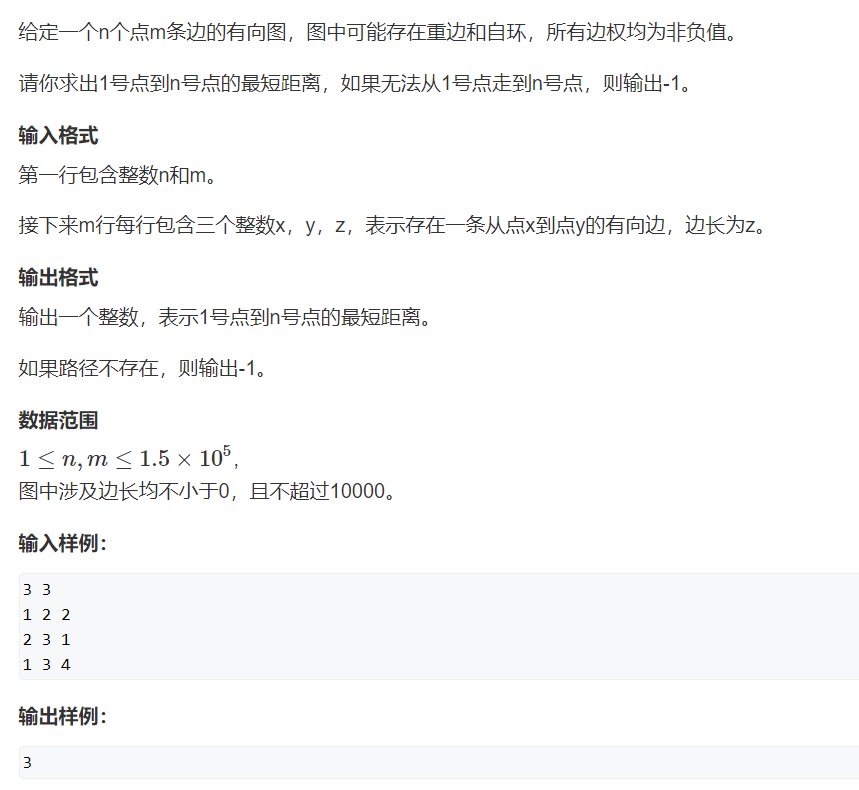#include <iostream>
#include <cstring>
#include <algorithm>
#include <queue>

using namespace std;

const int N = 2e5;

int n,m;
int h[N],w[N],e[N],ne[N],idx; //邻接表存储
int dist[N];
bool st[N];

typedef pair<int,int> PII; //first存距离，second存结点编号

{
e[idx] = b,w[idx] = c,ne[idx] = h[a], h[a] = idx ++ ;
}

int dijkstra()
{
//初始化
memset(dist,0x3f,sizeof(dist));
dist = 0;

priority_queue<PII,vector<PII>,greater<PII>> heap; //小根堆
heap.push({0,1});

while(heap.size())
{
PII t = heap.top();
heap.pop();

int ver = t.second, distance = t.first; //定义ver为编号，distance为距离
if(st[ver]) continue;
st[ver] = true;

for(int i = h[ver]; i != -1; i = ne[i]) //用t更新其他点的最短距离
{
int j = e[i];
if(dist[j] > distance + w[i])
{
dist[j] = distance + w[i];
heap.push({dist[j],j});
}
}
}
if(dist[n] == 0x3f3f3f3f) return -1;
return dist[n];
}

int main()
{
//初始化邻接表
memset(h,-1,sizeof h);
cin >> n >> m;
while(m -- )
{
int a,b,c;
scanf("%d%d%d",&a,&b,&c);
}

cout << dijkstra();

return 0;
}

Bellman-Ford算法
思路：循环n次，每次循环都遍历所有边，每次都更新一个结点的最短距离，那么循环n次，就可以确定n个点的最短路。 注：存在最短路时，一般不存在负权回路，即使存在，这个负环也不能在这条路径上（若存在负权回路，那么每在这个回路上转一圈，距离都会减小，这样可以无限次停留在负权回路，导致距离变为 - ∞） 实现步骤： 1.初始化： dist = 0，dist[i] = + ∞ 2.for循环n次： for循环m次：遍历所有边 dist[b] = dist[a] + w; （松弛条件） 时间复杂度O(nm) 循环n此后所有的边都满足 dist[b] ≤dist[a] + w （三角不等式） 迭代k次的含义：从1号点经过不超过k条边走到每个点的最短距离 找负环的方法：若果第n次迭代时，又更新了路径，说明存在长度≥n的最短路径。如果一个路径有n条边，那么就意味着有n+1个点，而图中一共只有n个点，由抽屉原理，就一定有两个点编号相同，那么这条路径上就一定存在环，而且是在更新过之后，所以这个环就一定是负环。所以这个算法可以用来找负环，方法就是看第n次是否有更新。（了解即可，一般找负环不用Bellman-Ford，而用SPFA）
典例——AcWing 853.有边数限制的最短路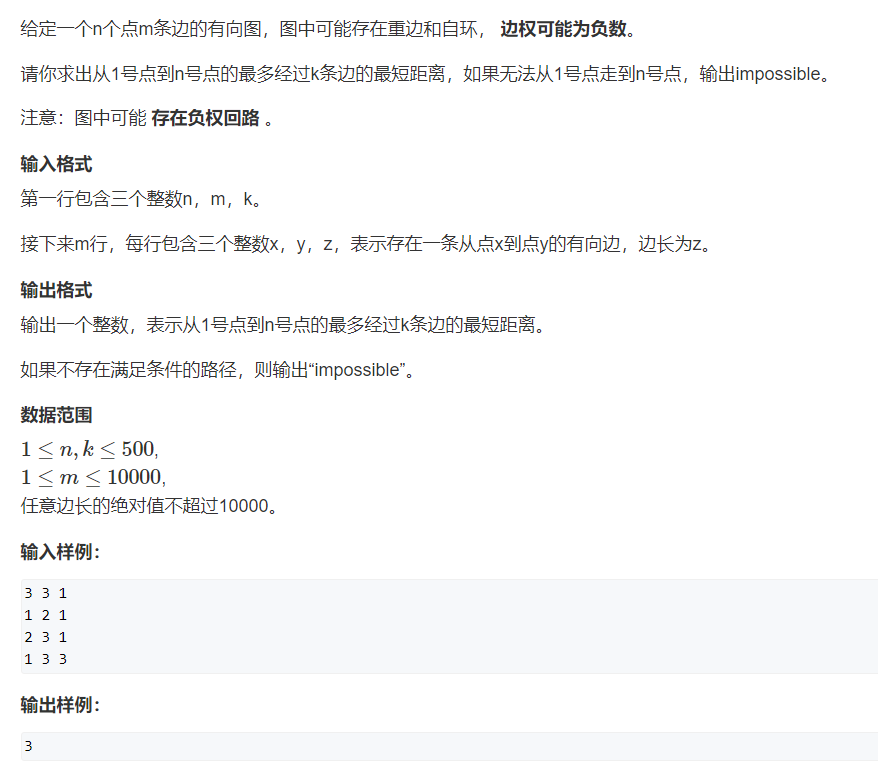#include <iostream>
#include <cstring>
#include <algorithm>

using namespace std;

const int N = 510,M = 10010;

int n,m,k;
int dist[N],backup[N]; //backup 为备份数组，存储dist上一次的值

struct edges
{
int a,b,w;
}edge[M];//存储边和权值

int bellman_ford()
{
//初始化
memset(dist,0x3f,sizeof(dist));
dist = 0;

//迭代k次
for(int i = 0;  i < k; i ++ )
{
memcpy(backup,dist,sizeof(dist)); //注1：备份

for(int j = 0; j < m; j ++ )
{
int a = edge[j].a, b = edge[j].b, w = edge[j].w;
dist[b] = min(dist[b],backup[a] + w); //用备份的值更新最短路
}
}

if(dist[n] > 0x3f3f3f3f / 2) return -1; //注2
return dist[n];
}
int main()
{
cin >> n >> m >> k;
for(int i = 0; i < m; i ++ )
{
int a,b,w;
cin >> a >> b >> w;
edge[i] = {a,b,w};
}

int t = bellman_ford();

if(t == -1) puts("impossible");
else cout << t << endl;

return 0;
}

思路解析： 这道题是Bellman-Ford算法的一个典型应用，而且此题只能用Bellman-Ford来做。 这道题的特殊性在于：要求的是从1号点到n号点最多经过k条边的最短距离，这里对边数做了限制，我们只需要迭代k此就可以了。 注1 memcpy(backup,dist,sizeof(dist))； 这里我们用backup数组来存储上一次迭代dist的值，防止发生串联，影响结果。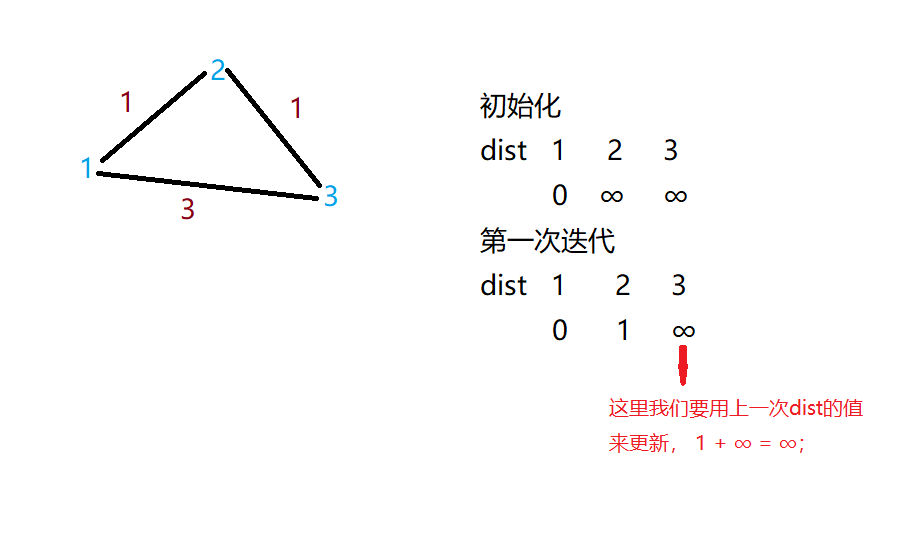注2 if(dist[n] > 0x3f3f3f3f / 2) return -1; 这里判断最短路不存在的条件为什么不能写成 if(dist[n] == 0x3f3f3f3f )呢？ 如果有负权边存在，我们在更新最短路的时候，可能会减掉一个数，∞减掉一个数，可能变成一个很大的而非∞的数，这时我们也认为，最短路是不存在的。
SPFA算法
SPFA适用于没有负环的图，但是绝大多数题目都是不存在负环的。SPFA算法是对上述Bellman-Ford算法的优化。Bellman-Ford是开两个循环，遍历每个点，每条边，这其中有很多重复的工作，SPFA对其进行了优化。 Bellman-Ford中dist[b] ≤dist[a] + w，dist[a]不一定是之前更新过的点，然而事实上，只有== 用之前被更新过的点去更新其他点，才会得到最短路 ==（只有一条路径上前面的点变小了，经过这个点的路径才会变小）。SPFA正是在这一点上进行了优化。 实现步骤：（用BFS来优化，队列中存储待更新的点）
1号点入队while(queue不空) ①取队头并入队（t = q.front() ） q.pop()； ②更新t的所有出边 t → b 更新成功后，如果b不在队中，则入队。（b→queue） SPFA算法实现的过程和Dijkstra算法实现过程非常相似。 典例——AcWing 851. spfa求最短路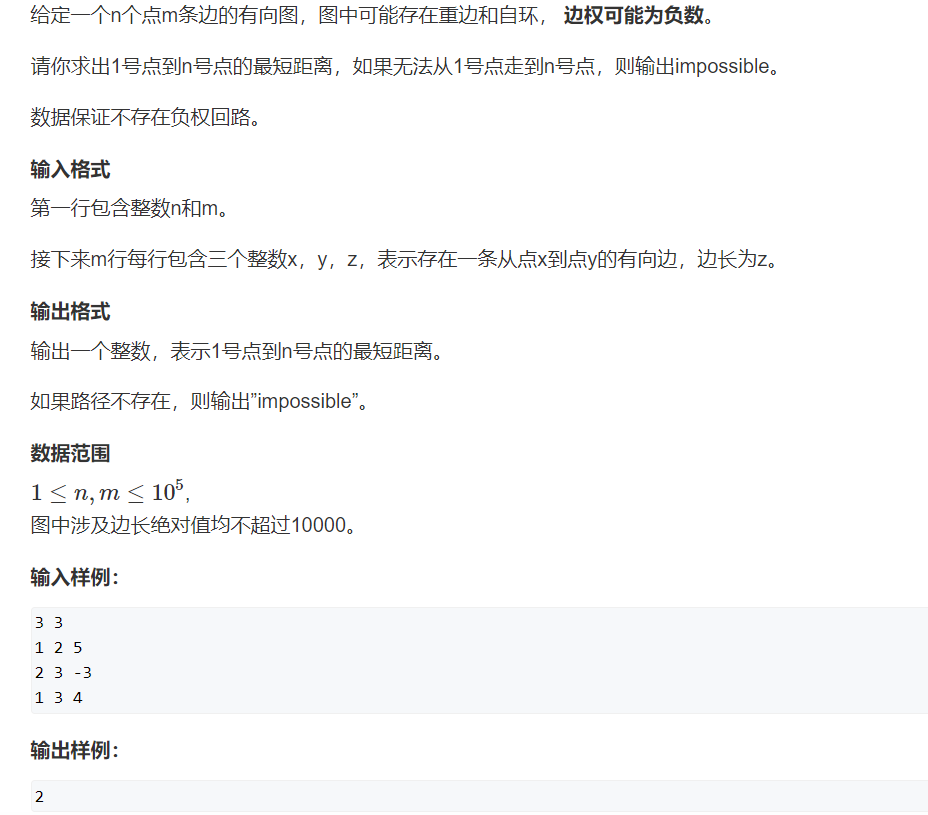#include <iostream>
#include <cstring>
#include <algorithm>
#include <queue>

using namespace std;

const int  N = 1e5 + 10,M = 1e5 + 10;

int n,m;
int h[N],e[N],w[N],ne[N],idx;
int dist[N];
bool st[N]; //标记第i个点是否在队列中，防止存储重复的点

{
e[idx] = b, w[idx] = c, ne[idx] = h[a], h[a] = idx ++ ;
}

int spfa()
{
memset(dist,0x3f,sizeof(dist));
dist = 0;

queue<int> q; //存储待更新的点
q.push(1); //1号点入队
st = true;

while(q.size()) //队列不空
{
int t = q.front(); //取队头
q.pop();
st[t] = false;

for(int i = h[t]; i != -1; i = ne[i]) //更新t的临边结点
{
int j = e[i];
if(dist[j] > dist[t] + w[i])
{
dist[j] = dist[t] + w[i];
if(!st[j])
{
q.push(j);
st[j] = true;
}
}
}
}
if(dist[n] == 0x3f3f3f3f) return -1;
return dist[n];
}
int main()
{
memset(h,-1,sizeof h);
cin >> n >> m;
while( m -- )
{
int a,b,c;
scanf("%d%d%d",&a,&b,&c);
}

int t = spfa();

if(t == -1) puts("impossible");
else
cout << t ;

return 0;

}

SPFA算法判断负环
思路与上面所说的Bellman-Ford算法判断负环是相同的，这里我们另开一个cnt数组来存当前最短路的边数。每次更新边的同时，更新cnt，当== cnt ≥ n ==时，就说明存在负环。（多出来的边一定是负环，不然不会被更新到最短路中）
典例——AcWing 852.spfa判断负环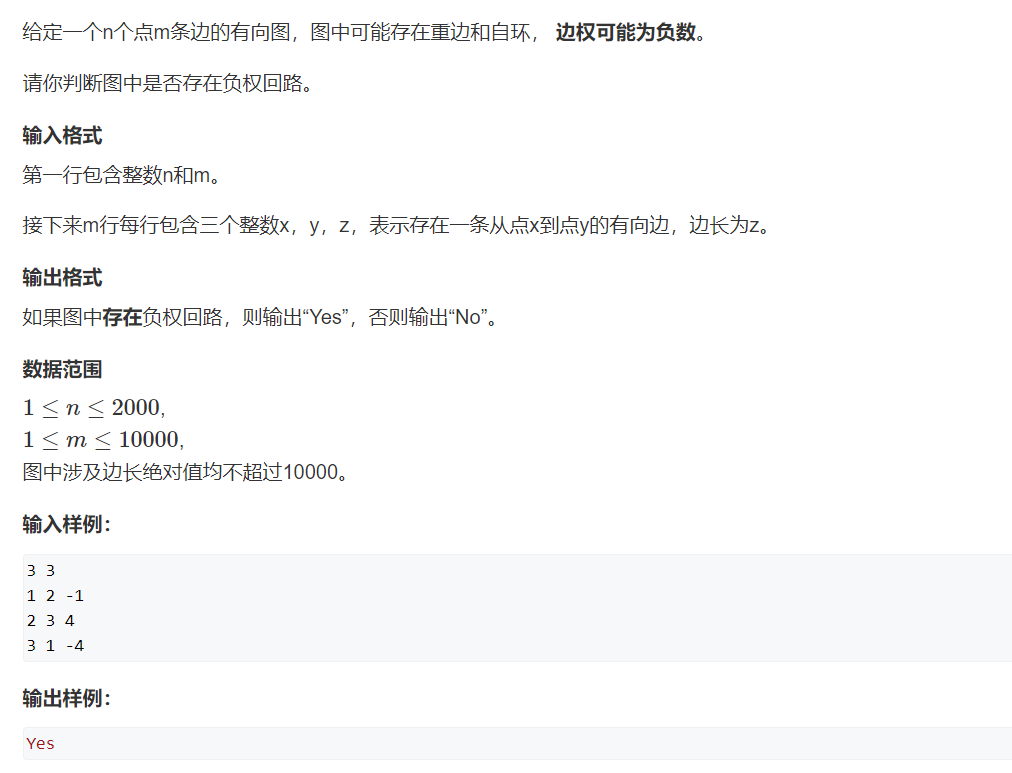#include <iostream>
#include <cstring>
#include <algorithm>
#include <queue>

using namespace std;

const int N = 2010,M = 10010;

int n,m;
int h[N],e[M],w[M],ne[M],idx;
int dist[M],cnt[M];
bool st[N];

{
e[idx] = b, w[idx] = c, ne[idx] = h[a], h[a] = idx ++ ;
}

int spfa()
{
queue<int> q;
//判断整个图中是否存在负环，需要将所有点入队
for(int i = 1; i <= n; i ++ )
{
q.push(i);
st[i] = true;
}

while(q.size())
{
int t = q.front();
q.pop();
st[t] = false;

for(int i = h[t]; i != -1; i = ne[i])
{
int j = e[i];
if(dist[j] > dist[t] + w[i])
{
dist[j] = dist[t] + w[i];
cnt[j] = cnt[t] + 1; //更新最短路上的边数

if(cnt[j] >= n) return true;
if(!st[j])
{
q.push(j);
st[j] = true;
}
}
}
}

return false;
}

int main()
{
cin >> n >> m;
memset(h,-1,sizeof h);
while(m -- )
{
int a,b,c;
cin >> a >> b >> c;
}
if(spfa()) puts("Yes");
else puts("No");

return 0;
}

多源汇最短路
Floyd算法
原理：基于动态规划，状态表示（三维）：d[k,i,j]：从点 i 只经过1~k这些中间点到达点 j 的最短距离。 那么就有：d[k,i,j] = d[k-1,i,k] + d[k-1,k,j] （状态更新） 思路：我们可以把点i到j的方式归为两类
从i 直接到 j；从i 经过 若干个点 到 j； 模板：三重循环，d(i,j)表示从i到j的最短路的长度
//初始化：
for (int i = 1; i <= n; i ++ )
for (int j = 1; j <= n; j ++ )
if (i == j) d[i][j] = 0; //Floyd算法要求不能存在负环
else d[i][j] = INF;
//算法实现
void floyd()
{
for (int k = 1; k <= n; k ++ )
for (int i = 1; i <= n; i ++ )
for (int j = 1; j <= n; j ++ )
d[i][j] = min(d[i][j], d[i][k] + d[k][j]);
}

典例——AcWing 854.Floyd 求最短路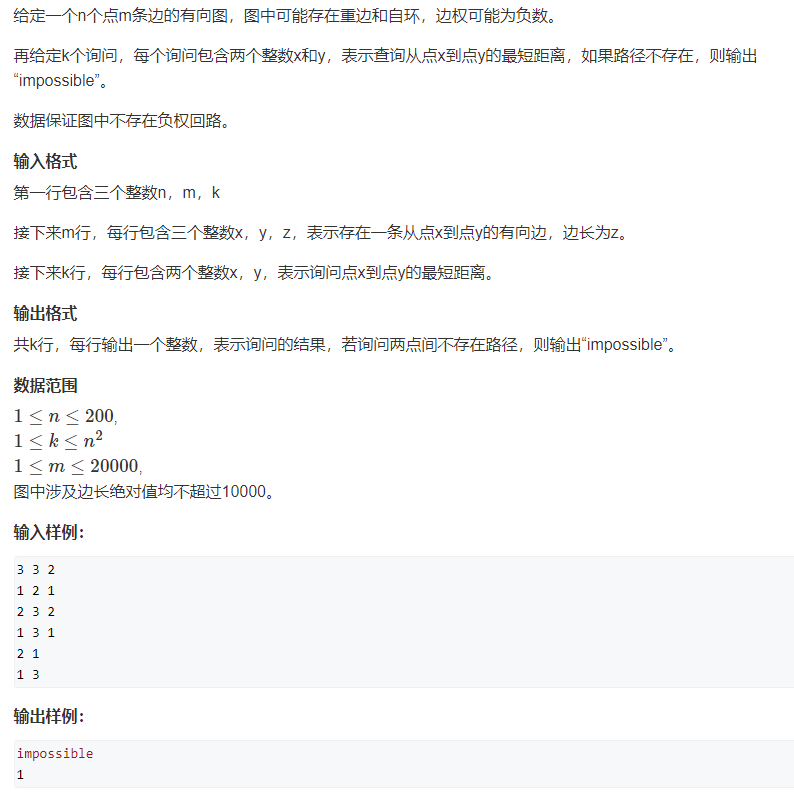#include <iostream>

using namespace std;

const int N = 210,M = 20010,INF = 1e9;

int n,m,k;
int d[N][N];

void floyd()
{
for(int k = 1; k <= n; k ++ )
for(int i = 1; i <= n; i ++ )
for(int j = 1; j <= n; j ++ )
d[i][j] = min(d[i][j],d[i][k] + d[k][j]);
}
int main()
{
cin >> n >> m >> k;
for(int i = 1; i <= n; i ++ )
for(int j = 1; j <= n; j ++ )
if(i == j)  d[i][j] = 0;
else d[i][j] = INF;

while(m -- )
{
int x,y,z;
cin >> x >> y >> z;

d[x][y] = min(d[x][y],z);
}

floyd();
while(k -- )
{
int x,y;
cin >> x >> y;

int t = d[x][y];
if(t > INF/2) puts("impossible");
//最短路不存在的判断条件写成t > INF/2，是因为可能存在负环，这个问题在前面有讲过。
else cout << t << endl;
}

return 0;
}

以上内容就是我总结的最短路问题的学习笔记。欢迎大佬们批评指正。
展开全文算法...